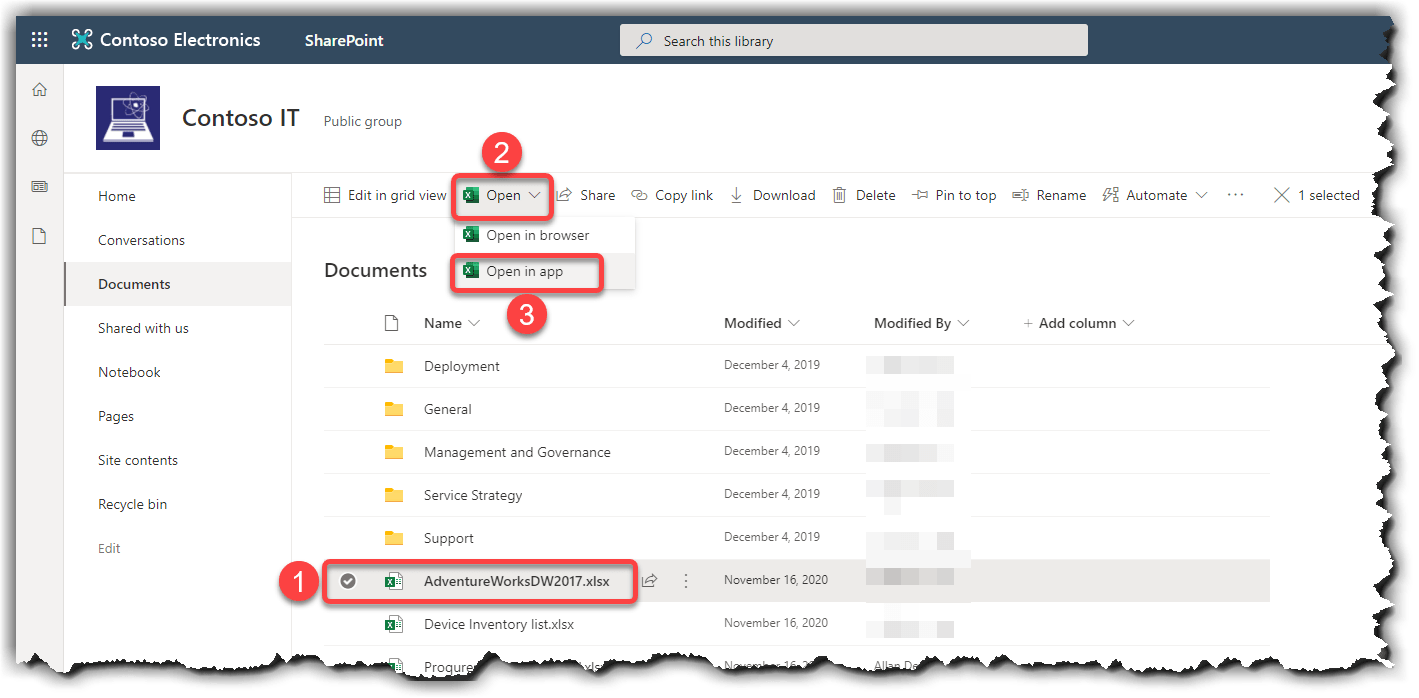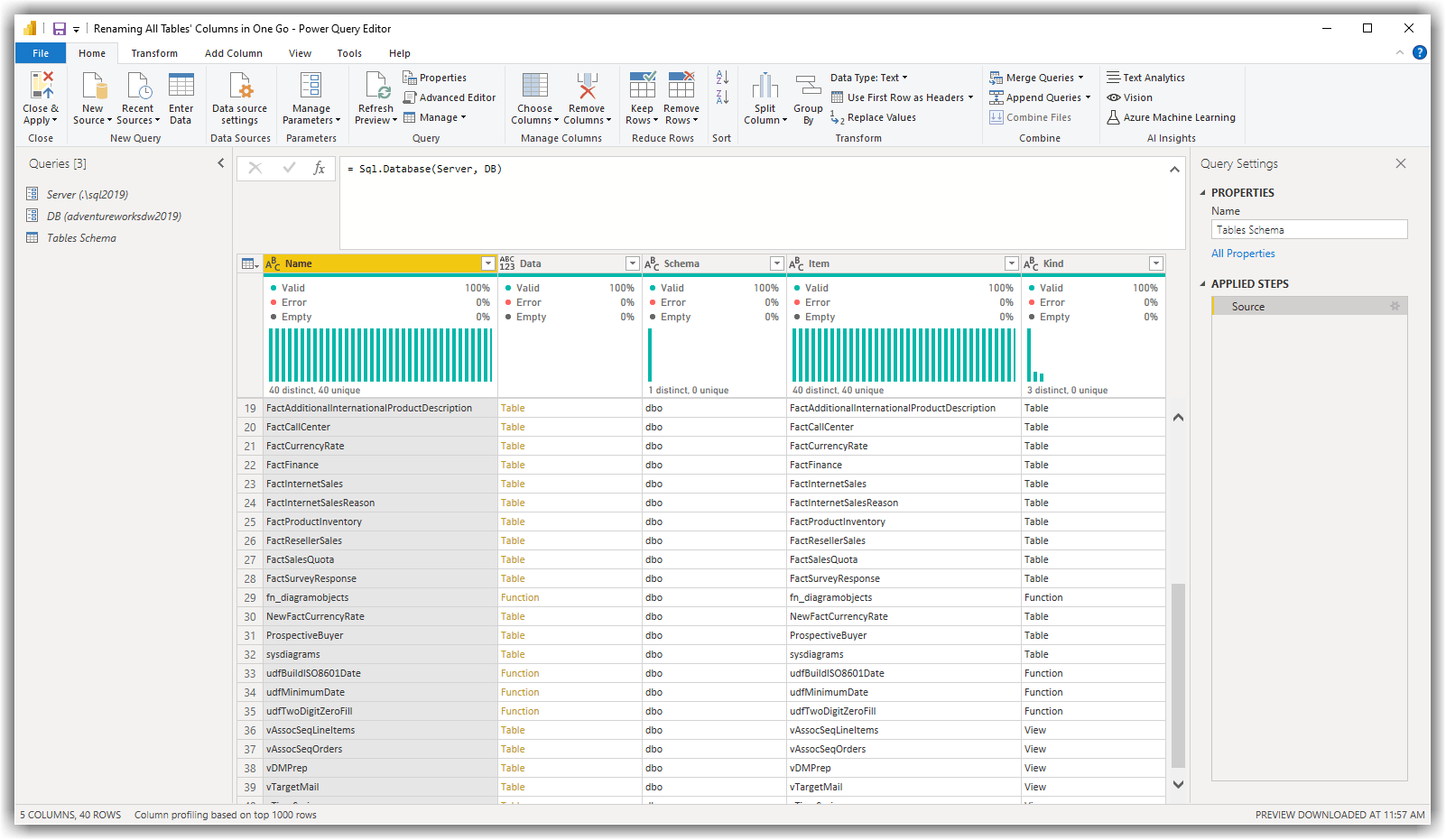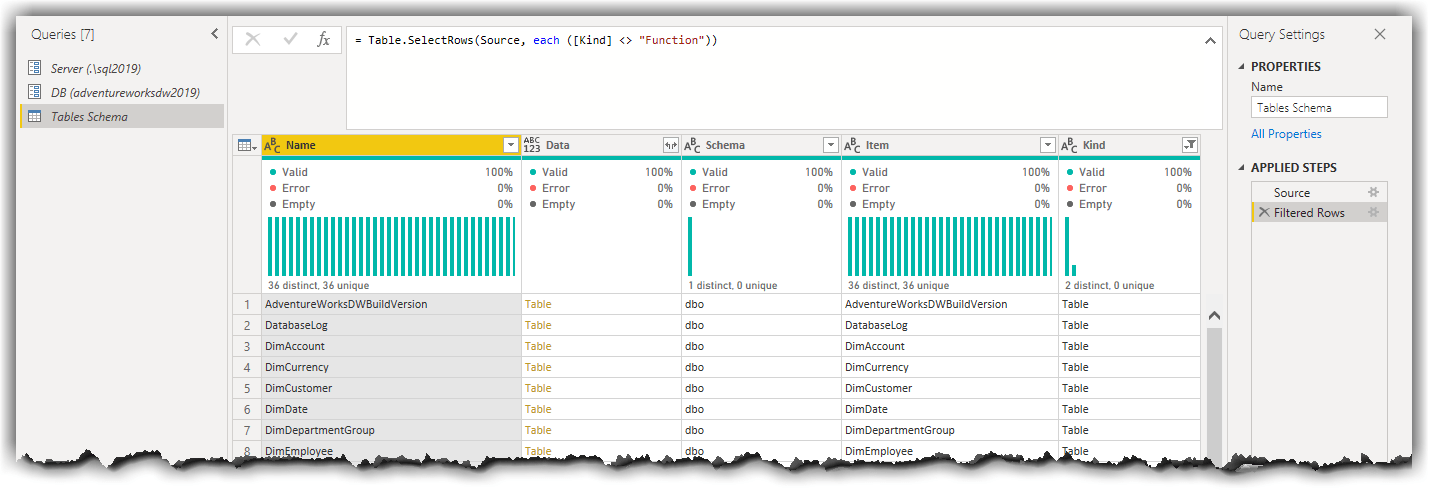## Quick Tips: Connecting to Excel Files Stored in SharePoint Online from Power BI Desktop

Microsoft Excel is one of the most common data sources for Power BI. We can store Excel files in various storage types. The way we get data from Excel varies depending on the storage type. In this post, I quickly show two methods to connect to an Excel file stored in SharePoint Online.

## Method 1: Getting the Excel File Path from the Excel Desktop App

This method requires you to have the Excel application installed on your machine. In this method, we open the Excel files stored in SharePoint Online in the Excel Desktop App in our machine and get the file path from there.

In SharePoint Online go to the desired document library then follow these steps to make it work:

1. Select the Excel file
2. Click the Open button
3. Click Open in appOpening Excel file from SharePoint Online in Excel Desktop App

This opens the Excel file in the Excel Desktop application. In the Excel follow these steps:

1. Click the File menu
2. Click Info
3. Click the Copy path buttonCopying Excel Path from Excel Desktop App

So far we got the Excel file path. The step is to get data from the copied path in Power BI Desktop.

Open Power BI Desktop and follow these steps:

1. Click Get data
2. Click WebGetting data from Excel stored in SharePoint Online in Power BI Desktop
1. Paste the path we copied from Excel in the URL text box
2. Delete the `?web=1` from the end of the copied path
3. Click OKModifying the path copied from Excel to get the data in Power BI Desktop
Continue reading “Quick Tips: Connecting to Excel Files Stored in SharePoint Online from Power BI Desktop”

## Quick Tips: Renaming All Tables’ Columns in One Go in Power Query

I previously wrote a blog post explaining how to rename all columns in a table in one go with Power Query. One of my visitors raised a question in the comments about the possibility to rename all columns from all tables in one go. Interestingly enough, one of my customers had a similar requirement. So I thought it is good to write a Quick Tip explaining how to meet the requirement.

## The Problem

You are connecting to the data sources from Power BI Desktop (or Excel or Data Flows). The columns of the source tables are not user friendly, so you require to rename all columns. You already know how to rename all columns of a table in one go but you’d like to apply the renaming columns patterns to all tables.

## The Solution

The solution is quite simple. We require to connect to the source, but we do not navigate to any tables straight away. In my case, my source table is an on-premises SQL Server. So I connect to the SQL Server instance using the `Sql.Database(Server, DB)` function in Power Query where the Server and the DB are query parameters. Read more about query parameters here. The results would like the following image:The results of running the Sql.Database(Server, DB) function

As you see in the above image, the results include Tables, Views and Functions. We are not interested in Functions therefore we just filter them out. The following image shows the results after applying the filter:Filtering out SQL Server Functions

If we look closer to the Data column, we see that the column is indeed a Structured Column. The structured values of the Data column are Table values. If we click on a cell (not on the Table value of the cell), we can see the actual underlying data, as shown in the following image:

Continue reading “Quick Tips: Renaming All Tables’ Columns in One Go in Power Query”

## Quick Tips: OData Feed Analyser Custom Function in Power Query

It’s been a while that I am working with OData data source in Power BI. One challenge that I almost always do not have a good understanding of the underlying data model. It can be really hard and time consuming if there is no one in the business that understands the underlying data model. I know, we can use `\$metadata` to get the metadata schema from the OData feed, but let’s not go there. I am not an OData expert but here is the thing for someone like me, I work with various data sources which I am not necessarily an expert in, but I need to understand what the entities are, how they are connected etc… then what if I do not have access any SMEs (Subject Matter Expert) who can help me with that?

So getting involved with more OData options, let’s get into it.

The custom function below accepts an OData URL then it discovers all tables, their column count, their row count (more on this later), number and list of related tables, number and list of columns of `type text`, `type number` and `Decimal.Type`.

``````// fnODataFeedAnalyser
(ODataFeed as text) =>
let
Source = OData.Feed(ODataFeed),
SourceToTable = Table.RenameColumns(
Table.DemoteHeaders(Table.FromValue(Source)),
{{"Column1", "Name"}, {"Column2", "Data"}}
),
FilterTables = Table.SelectRows(
SourceToTable,
each Type.Is(Value.Type([Data]), Table.Type) = true
),
SchemaAdded = Table.AddColumn(FilterTables, "Schema", each Table.Schema([Data])),
TableColumnCountAdded = Table.AddColumn(
SchemaAdded,
"Table Column Count",
each Table.ColumnCount([Data]),
Int64.Type
),
TableCountRowsAdded = Table.AddColumn(
TableColumnCountAdded,
"Table Row Count",
each Table.RowCount([Data]),
Int64.Type
),
NumberOfRelatedTablesAdded = Table.AddColumn(
TableCountRowsAdded,
"Number of Related Tables",
each List.Count(Table.ColumnsOfType([Data], {Table.Type}))
),
ListOfRelatedTables = Table.AddColumn(
NumberOfRelatedTablesAdded,
"List of Related Tables",
each
if [Number of Related Tables] = 0 then
null
else
Table.ColumnsOfType([Data], {Table.Type}),
List.Type
),
NumberOfTextColumnsAdded = Table.AddColumn(
ListOfRelatedTables,
"Number of Text Columns",
each List.Count(Table.SelectRows([Schema], each Text.Contains([Kind], "text"))[Name]),
Int64.Type
),
ListOfTextColunmsAdded = Table.AddColumn(
NumberOfTextColumnsAdded,
"List of Text Columns",
each
if [Number of Text Columns] = 0 then
null
else
Table.SelectRows([Schema], each Text.Contains([Kind], "text"))[Name]
),
NumberOfNumericColumnsAdded = Table.AddColumn(
ListOfTextColunmsAdded,
"Number of Numeric Columns",
each List.Count(Table.SelectRows([Schema], each Text.Contains([Kind], "number"))[Name]),
Int64.Type
),
ListOfNumericColunmsAdded = Table.AddColumn(
NumberOfNumericColumnsAdded,
"List of Numeric Columns",
each
if [Number of Numeric Columns] = 0 then
null
else
Table.SelectRows([Schema], each Text.Contains([Kind], "number"))[Name]
),
NumberOfDecimalColumnsAdded = Table.AddColumn(
ListOfNumericColunmsAdded,
"Number of Decimal Columns",
each List.Count(
Table.SelectRows([Schema], each Text.Contains([TypeName], "Decimal.Type"))[Name]
),
Int64.Type
),
ListOfDcimalColunmsAdded = Table.AddColumn(
NumberOfDecimalColumnsAdded,
"List of Decimal Columns",
each
if [Number of Decimal Columns] = 0 then
null
else
Table.SelectRows([Schema], each Text.Contains([TypeName], "Decimal.Type"))[Name]
),
#"Removed Other Columns" = Table.SelectColumns(
ListOfDcimalColunmsAdded,
{
"Name",
"Table Column Count",
"Table Row Count",
"Number of Related Tables",
"List of Related Tables",
"Number of Text Columns",
"List of Text Columns",
"Number of Numeric Columns",
"List of Numeric Columns",
"Number of Decimal Columns",
"List of Decimal Columns"
}
)
in
#"Removed Other Columns"``````
Continue reading “Quick Tips: OData Feed Analyser Custom Function in Power Query”

## Finding Minimum Date and Maximum Date Across All Tables in Power Query in Power BI and Excel

When we talk about data analysis in Power BI, creating a Date table is inevitable. There are different methods to create a Date table either in DAX or in Power Query. In DAX you my use either `CALENDAR()` function or `CALENDARAUTO()` function to create the Date table. In Power Query you may use a combination of `List.Dates()``#date()` and `#duration()` functions. Either way, there is one point that is always challenging and it is how to find out a proper date range, starting from a date in the past and ending with a date in the future, that covers all relevant dates within the data model. One simple answer is, we can ask the business. The SMEs know what the valid date range is..

While this is a correct argument it is not always the case. Especially with the Start Date which is a date in the past. In many cases the business says:

Lets’s have a look at the data to find out.

That is also a correct point, we can always a look at the data, find all columns with either `Date` or `DateTime` datatypes then sort the data in ascending or descending order to get the results. But what if there many of them? Then this process can be very time consuming.

Many of you may already thought that we can use `CALENDARAUTO()` in DAX and we are good to go. Well, that’s not quite right. In many cases there are some `Date` or `DateTime` columns that must not be considered in our Date dimension. Like Birth Date or Deceased Date. More on this later in this post.

In this post I share a piece of code I wrote for myself. I was in a situation to identify the Start Date and the End Date of the date dimension many times, so I thought it might help you as well.

# How it works?

The Power Query expressions I share in this post starts with getting all existing queries using:

• `#sections` intrinsic variable
• Filtering out the current query name, which is GetMinMaxAllDates in my sample, to avoid getting the following error:

Expression.Error: A cyclic reference was encountered during evaluation.

Continue reading “Finding Minimum Date and Maximum Date Across All Tables in Power Query in Power BI and Excel”